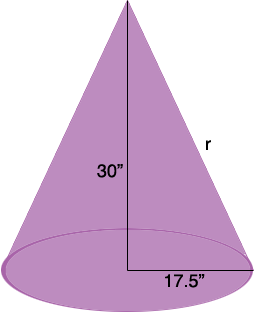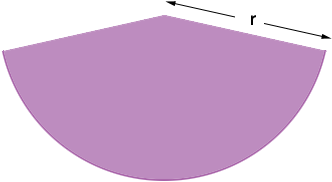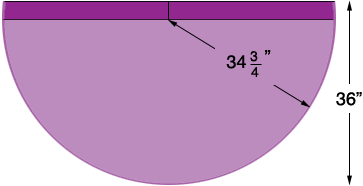SEARCH HOMEMath Central Quandaries & QueriesQuestion from Susan, a parent: Hi!!! I am making a play tent as seen at the link below and need to figure out how to get the dimensions for the cone shape. The one shown has 4 different seams, but I guess I can get away with just one seam to sew it together (?) I need it to go over a hula hoop as that is what I am using for the round support at the top. My hula hoop is 35" in diameter from outside edge to outside edge. I would like the height of the teepee to be around 30" from the center to the peak. Thanks so much, oh my gosh, I have been fretting for 2 days about this and my hair is about to fall out!!!! Please contact me if you need any additional info. ~Susan http://www.landofnod.com/family.aspx?c=52&f=4100Hi Susan,

Cute project!

Here is a sketch of the cone shaped top.You have a right triangle with legs of length 17.5" and 30" and hypotenuse of length r inches so Pythagoras theorem says that

17.52 + 302 = r2

or

r = 34.7 inches.

If you took the material from the cone in the diagram and cut it along one of the seams it will then lie flat in the shape of a sector of a circle as in the diagram below.The circumference of the hula hoop is π × diameter = π × 35 = 109.0 inches which is the length of the arc in the sector above. This is a sector of a circle with radius 34.7 inches or diameter 69.4 inches so the circumference of this circle is π × diameter = π × 69.4 = 218.0 inches. Thus the arc length is half the circumference and hence the sector is a semicircle.

Hence if you cut a semicircle of radius 34.7 inches which is about 34 3/4 inches it will form the cone you need. Unfortunately this leaves no material for a seam. If your material is 36 inches wide I would cut a piece like thisleaving you an inch and a quarter for seams.

If you complete this project send us a photo so we can post it.

PennyMath Central is supported by the University of Regina and The Pacific Institute for the Mathematical Sciences.